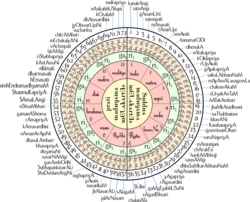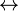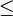## Katapayadi SankhyaKatapayadi sankhya(Sanskrit: कटपयादि  system is from Grahacāraṇibandhana by Haridatta in 683 CE. It has been used in Laghu·bhāskarīya·vivaraṇa written by Śaṅkara·nārāyaṇa in 869 CE..It   It is a simplification of Aryabhata‘s Sanskrit numerals , due probably to Haridatta from Kerala. In Malayalam it is also known as ‘Paralperu’ For eg:ചണ്ഡാംശുചന്ദ്രാധമകുംഭിപാല represents 31415926536 which isπ*1000000000000000 .

## Rules and practices

Following verse found in Śaṅkaravarman's Sadratnamāla explains the mechanism of the system.
नज्ञावचश्च शून्यानि संख्या: कटपयादय:।
मिश्रे तूपान्त्यहल् संख्या न च चिन्त्यो हलस्वर:॥
Transiliteration:
nanyāvacaśca śūnyāni saṃkhyāḥ kaṭapayādayaḥ
miśre tūpāntyahal saṃkhyā na ca cintyo halasvaraḥ
Translation: na (न), nya (ञ) and a (अ)-s, i.e., vowels represent zero. The nine integers are represented by consonant group beginning with ka, ṭa, pa, ya. In a conjunct consonant, the last of the consonants alone will count. A consonant without vowel is to be ignored.
Explanation: The assignment of letters to the numerals are as per the following arrangement.
1234567890
ka क క കkha ख ఖ ഖga ग గ ഗgha घ ఘ ഘnga ङ జ్ఞ ങca च చ ചcha छ ఛ ഛja ज జ ജjha झ ఝ ഝnya ञ ఞ ഞ
ṭa ट ట ടṭha ठ ఠ ഠḍa ड డ ഡḍha ढ ఢ ഢṇa ण ణ ണta त త തtha थ థda द ద ദdha ध ధ ധna न న ന
pa प ప പpha फ ఫ ഫba ब బ ബbha भ భ ഭma म మ മ-----
ya य య യra र ర രla ल ల ലva व వ വśha श శ ശsha ष ష ഷsa स స സha ह హ ഹ--
• Consonants have numerals assigned as per the above table. For example, ba (ब) is always 3 whereas 5 can be represented by either nga (ङ) or ṇa (ण) or ma (म) or śha (श).
• All stand-alone vowels like a (अ) and (ऋ) are assigned to zero.
• In case of a conjunct, consonants attached to a non-vowel will not be valueless. For example, kya (क्या) is formed by k (क्) + ya (य) + a (अ). The only consonant standing with a vowel is ya (य). So the corresponding numeral for kya (क्या) will be 1.
• There is no way of representing Decimal separator in the system.
• Indians used the Hindu-Arabic numeral system for numbering, traditionally written in increasing place values from left to right. This is as per the rule aṅkānām vāmato gatiḥ (अङ्कानाम् वामतो गतिः) which means numbers go from left to right.
• ## Usage

### Mathematics and astronomy

• Karaṇa·paddhati, written in the 15th century, has the following śloka for the value of pi (π)
അനൂനനൂന്നാനനനുന്നനിത്യൈ-
സ്സമാഹതാശ്ചക്രകലാവിഭക്താഃ
ചണ്ഡാംശുചന്ദ്രാധമകുംഭിപാലൈര്‍-
വ്യാസസ്തദര്‍ദ്ധം ത്രിഭമൗര്‍വിക സ്യാത്‌
Transliteration
anūnanūnnānananunnanityai
ssmāhatāścakra kalāvibhaktoḥ
caṇḍāṃśucandrādhamakuṃbhipālair
vyāsastadarddhaṃ tribhamaurvika syāt
It gives the circumference of a circle of diameter, anūnanūnnānananunnanityai (10,000,000,000) as caṇḍāṃśucandrādhamakuṃbhipālair (31415926536).
भद्राम्बुद्धिसिद्धजन्मगणितश्रद्धा स्म यद् भूपगी:
Transliteration
bhadrāṃbuddhisiddhajanmagaṇitaśraddhā sma yad bhūpagīḥ
Splitting the consonants gives,
भ bhaद् dरा rāम् ṃबु baद् dधि dhसि saद् dध dhaज jaन् nम maग gaणि ṇaत taश् ṣर raद् dधा dhaस् sम maय yaद् dभू bhaप paगि gi
4-2-3-97-98-5356-2-9-51-413
Reversing the digits to modern day usage of descending order of decimal places, we get 314159265358979324 which is the value of pi (π) to 17 decimal places, except the last digit might be rounded off to 4.
• This verse encrypts the value of pi (π) up to 31 decimal places.
``` गोपीभाग्यमधुव्रात-श्रुग्ङिशोदधिसन्धिग ॥
खलजीवितखाताव गलहालारसंधर ॥
```
``` ಗೋಪೀಭಾಗ್ಯಮಧುವ್ರಾತ-ಶ್ರುಂಗಿಶೋದಧಿಸಂಧಿಗ ||
ಖಲಜೀವಿತಖಾತಾವ ಗಲಹಾಲಾರಸಂಧರ ||
```
This verse directly yields the decimal equivalent of pi divided by 10: pi/10 = 0.31415926535897932384626433832792
``` గోపీభాగ్యమధువ్రాత-శృంగిశోదధిసంధిగ |
ఖలజీవితఖాతావ గలహాలారసంధర ||
```

#### Music Use-(FROM MYSTERIESEXPLORED)

The “ka-ta-pa-ya-di” rule used by ancient Indian mathematicians and grammarians is a tool to map names to numbers. Writing the consonants of the sanskrit alphabet as four groups with “Ka, Ta, Pa, Ya” as the begining letters of the groups we getEach letter of the group is numbered from 1 through 9 and 0 for the tenth letter. Thus, ka is 1, sa is 7, ma is 5, na is 0 and so on. So to indicate the number 356 for example one would try and come up with a word involving the third, fifth and sixth letters of the groups like “gaNitam” or “lESaca”.
However, in the Indian tradition, the digits of a number are written left to right in the increasing order of their place value – exactly opposite the way we are used to writing in the western way. Therefore 356 would be indicated using letters in the 6th, 5th, and 3rd positions of the group e.g. “triSUlaM”.
Here is an actual verse of spiritual content, as well as secular mathematical significance:
“gopi bhagya madhuvrata
srngiso dadhi sandhiga
khala jivita khatava
gala hala rasandara”
The translation is as follows: “O Lord anointed with the yoghurt of the milkmaids’ worship (Krishna), O savior of the fallen, O master of Shiva, please protect me.”
Vowels make no difference and it is left to the author to select a particular consonant or vowel at each step. This great latitude allows one to bring about additional meanings of his choice. For example kapa, tapa, papa, and yapa all mean 11.
Now the interesting fact is that when you start numbering the consonants with the respective numbers from go = 3, pi = 1, bha =4 , ya = 1 , ma = 5 , duv = 9 and so on. you will end with the number 31415926535897932384626433832792.

This is the decimal equivalent of the ratio of the circumference of a circle to its diameter, Which you IS “pi”. The above number gives the accurate value of pi/10 correct to 31 decimal places.
Also not only did the code give pi up to 32 decimal places , but there was a secret Master key within the patterning of the 32 that could unlock the next 32 decimals of the pi, and so on. A trick to infinity.
The Code not only praised Krishna, it operated on another level as a dedication to Lord Shankara or Shiva.
In 825 AD one Arab mathematician
said: “This value has been given by the Hindus [Indians]”
Another amazing fact is that…
Shanu A. from Kerela has recently entered the Guiness Book of Records for his feat of memorising the value of Pi upto 50,000 places. He says he has been inspired by the Vedic numerical code which he used to memorise the entire value of Pi. He used the Vedic Numerical Code innovatively as a peg system and improvised on it. That was his secret.

### Carnatic music-

• The melakarta ragas of the Carnatic music is named so that the first two syllables of the name will give its number. This system is sometimes called the Ka-ta-pa-ya-di sankhya. The Swaras 'Sa' and 'Pa' are fixed, and here is how to get the other swaras from the melakarta number.
1. Melakartas 1 through 36 have Ma1 and those from 37 through 72 have Ma2.
2. The other notes are derived by noting the (integral part of the) quotient and remainder when one less than the melakarta number is divided by 6.
3. 'Ri' and 'Ga' positions: the raga will have:
• Ri1 and Ga1 if the quotient is 0
• Ri1 and Ga2 if the quotient is 1
• Ri1 and Ga3 if the quotient is 2
• Ri2 and Ga2 if the quotient is 3
• Ri2 and Ga3 if the quotient is 4
• Ri3 and Ga3 if the quotient is 5
4. 'Da' and 'Ni' positions: the raga will have:
• Da1 and Ni1 if remainder is 0
• Da1 and Ni2 if remainder is 1
• Da1 and Ni3 if remainder is 2
• Da2 and Ni2 if remainder is 3
• Da2 and Ni3 if remainder is 4
• Da3 and Ni3 if remainder is 5
• #### Raga Dheerasankarabharanam

The katapayadi scheme associates dha$\leftrightarrow$9 and ra$\leftrightarrow$2, hence the raga's melakarta number is 29 (92 reversed). Now 29$\le$ 36, hence Dheerasankarabharanam has Ma1. Divide 28 (1 less than 29) by 6, the quotient is 4 and the remainder 4. Therefore, this raga has Ri2, Ga3 (quotient is 4) and Da2, Ni3 (remainder is 4). Therefore, this raga's scale is Sa Ri2 Ga3 Ma1 Pa Da2 Ni3 SA.

#### Raga MechaKalyani

From the coding scheme Ma$\leftrightarrow$ 5, Cha$\leftrightarrow$ 6. Hence the raga's melakarta number is 65 (56 reversed). 65 is greater than 36. So MechaKalyani has Ma2. Since the raga's number is greater than 36 subtract 36 from it. 65-36=29. 28 (1 less than 29) divided by 6: quotient=4, remainder=4. Ri2 Ga3 occurs. Da2 Ni3 occurs. So MechaKalyani has the notes Sa Ri2 Ga3 Ma2 Pa Da2 Ni3 SA.

#### Exception for Simhendramadhyamam

As per the above calculation, we should get Sa$\leftrightarrow$ 7, Ha$\leftrightarrow$ 8 giving the number 87 instead of 57 for Simhendramadhyamam. This should be ideally Sa$\leftrightarrow$ 7, Ma$\leftrightarrow$ 5 giving the number 57. So it is believed that the name should be written as Sihmendramadhyamam (as in the case of Brahmana in Sanskrit).

• ### mysteriesexplored.wordpress.com

#### 2 comments:

1.2.What is katapayathi number of pramesh